# Module FreeVerticalMovement

## Overview

The free vertical movement module computes particulate water properties vertical fluxes. It is normally used to computed settling velocity in cohesive sediment or particulate organic matter transport simulations.

## Equations

### Constant settling velocity

Two different approaches are followed to compute settling velocity: a constant settling velocity and a cohesive sediment concentration dependent settling velocity. In the first case, each particulate property can have its specific and constant settling velocity. This is considered to be a reasonable approach for free settling concentrations ranges.

### Cohesive sediment concentration dependent settling velocity

Formulation used in Mohid regards only flocculation and hindered settling concentration ranges. The general correlation for the settling velocity in the flocculation range is: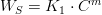$W_S = K_1 \cdot C^m$ for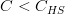$C < C_{HS}$

and in the hindered settling range is: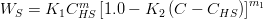$W_S = K_1 C_{HS} ^m \left[ {1.0 - K_2 \left( {C - C_{HS} } \right)} \right]^{m_1 }$ for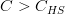$C > C_{HS}$

where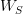$W_S$ in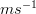$m s^{-1}$ is the settling velocity, C (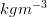$kg m^{-3}$) is the cohesive sediment concentration, and the subscript HS refers to the onset of the hindered settling (of about 2 to 5$kg m^{-3}$). The coefficients K1 (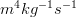$m^{4}kg^{-1}s^{-1}$) and K2 (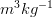$m^{3}kg^{-1}$) depend on the mineralogy of the mud and the exponents m and m1 depend on particle size and shape.

### Flocculation

Flocculation can be parameterized in MOHID by defining a limit for the salinity concentration above which flocculation is considered to be complete. Below this limit there is no flocculation, thus the settling velocity is null. This process can be optionally activated in the model and it can be used along with a constant settling velocity definition or a cohesive sediment concentration dependent settling velocity.